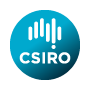# Software from Alan J. Miller

 Index Applied statistics algorithms Quadruple precision code (F) Quadruple precision Random Toms

## Some Applied Statistics Algorithms

• as6.f90 Cholesky factorization of symmetric positive definite matrix with only lower triangle stored as a vector (2 versions).
• as7.f90 Inversion of symmetric positive definite matrix with only lower triangle stored as a vector using AS6 (2 versions). Includes short test program and interfaces.
• as27.f90 Upper tail area under Student's t-distribution.
• as60.f90 Calculates the eigenvalues/vectors of a real symmetric matrix.
• as63.f90 Computes the incomplete beta function. The file includes a module for the log of the beta function, which also has the log of the gamma function.
• as66.f90 Area under the normal curve.
• as91.f90 Returns percentage points of the chi-squared distribution for input tail probabilities and numbers of degrees of freedom.
• as110 Lp-norm fitting of straight lines, particularly for 1 <= p <= 2, by extension of an algorithm of Schlossmacher.
• chirp.f90 The CHIRP-Z Fast Fourier transform for series of arbitrary length. Refer to the journal article for instructions on its use. Includes FFT for the usual case when series length is a power of 2. this is AS120.
• as126 Calculates the distribution function for the range for a sample of size n from the standard normal distribution.
• as132.f90 Minimize the sum of absolute deviations for a simple Y on X linear regression.
• as135.f90 Min-Max (L-infinity) estimates for linear multiple regression, includes a test program.
• as136.f90 A K-means clustering algorithm.
• as152.f90 Cumulative hypergeometric probabilities.
• as154.f90 Combines AS 154 & 182, for the exact maximum likelihood estimation of parameters of ARIMA models using Kalman filtering.
• as155.f90 Distribution of a linear combination of non-central chi-squared random variables. This code is NOT compatible with Lahey's ELF90 compiler.
• as157.f90 The runs-up and runs-down tests.
• as177.f90 Calculates the expected values of normal order statistics.
• as181.f90 Calculates the Shapiro-Wilks statistic and its P-value. Actually uses ASR94.
• as190.f90 Distribution function and its inverse, for the studentized range.
• as192.f90 Computes approximate significance points of a Pearson curve with given first four moments, or first three moments and left or right boundary.
• as205.f90 Enumeration of all R x C contingency tables with given row and column totals, and calculation of hypergeometric probability for each table.
• as207.f90 Fits a general log-linear model. N.B. The F90 version has NOT been tested by me (Alan Miller).
• as217.f90 Does the dip calculation for an ordered vector X using the greatest convex minorant and the least concave majorant, skipping through the data using the change points of these distributions. It returns the dip statistic 'DIP' and the modal interval (XL, XU).
• as227.f90 Generates all possible N-bit binary codes.
• as239.f90 Integral of the gamma distribution
• as241.f90 Calculate the normal deviate (z-score) corresponding to a given area under the normal curve
• as245.f90 Log of the gamma function
• as260.f90 Calculates the distribution function of the square (R^2) of the multiple correlation coefficient.
• as261.f90 Quantiles of the distribution of R^2.
• as275.f90 Calculation of the non-central chi-squared distribution function.
• as282.f90 Robust regression using the least median of squares (LMS) criterion. There is also a test program: t_as282.f90
• as285.f90 Multivariate normal probabilities over regions defined in a user-supplied function.
• as286.zip Parameter estimation for non-linear errors-in-variables problems. I have added a third example, fitting a cylinder, to the two which were in the published version. This is a ZIP file.
• as290.f90 Generates a rectangular grid which can be used for contour plotting of confidence limits in nonlinear regression. Also outputs the F-values for the confidence levels selected. The test program: t_as290.f90 produces the grid for the beanroot data used in the paper in Applied Statistics: beanroot.dat
• as295.f90 Algorithm for generating designs of experiments which are close to D-optimal. The user supplies the set of NCAND candidate design points and the algorithm picks a subset of N of them. Tha main uses of this algorithm are likely to be for choosing designs in situations in which there are blocks of an awkward size, and for augmenting an experiment which has already been carried out. Two driver programs are provided here: fr_fac23.f90 for 2^p x 3^q fractional factorial experiments with possibly unequal block sizes, and ibquad.f90 for quadratic surface designs with possibly unequal block sizes.
• as298.f90 Hybrid minimization routine using simulated annealing and a user-supplied local minimizer. You should also look at Dr. Tibor Csendes' global optimization package here.
• as304.f90 Fisher's non-parametric randomization test for two small independent random samples.
• as310.f90 Incomplete beta function.
• as319.f90 Variable metric unconstrained function minimization without derivatives.